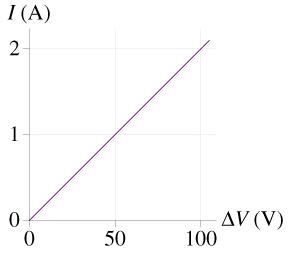# Problem: The figure is a current-versus-potential-difference graph for a cylinder.What is the cylinder's resistance?

###### FREE Expert Solution

Ohm's law:

$\overline{){\mathbf{V}}{\mathbf{=}}{\mathbf{I}}{\mathbf{R}}}$

R = SLOPE = ΔV/Δi

Using the points: (100V,2A) and (0V,0A)

90% (364 ratings)###### Problem DetailsThe figure is a current-versus-potential-difference graph for a cylinder.

What is the cylinder's resistance?